# Latex常规环境中的常用语句

### 1. 空格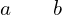—— a \qquad b % 两个m的宽度（两个quad空格）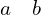—— a \quad b % 一个m的宽度（quad空格）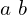—— a\ b % 1/3m宽度（大空格）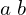—— a\;b % 2/7m宽度（中等空格）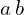—— a\,b % 1/6m宽度（小空格）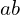—— ab % 没有空格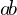—— a\!b % 缩进1/6m宽度（紧贴）

### 2. 换行

\\

### 3. 居中

\begin{center}  \end{center}

### 4. 图片

\usepackage{graphicx}

\begin{figure}[htbp]
\includegraphics*[width=0.5\textwidth]{a.eps}
\caption{This is a caption.}
\label{fig:a}
\end{figure}

### 5. 表格

\begin{table}[htbp]
\begin{tabular}{| c | c | c |}
\hline
a & b & c \\
\hline
1 & 2 & 3 \\
\hline
4 & 5 & 6  \\
\hline
7 & 8 & 9  \\
\hline
\end{tabular}
\caption{This is a caption.}
\label{table:a}
\end{table}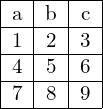### 6. 超链接

\usepackage{hyperref}

\hypersetup{
anchorcolor=blue
filecolor=blue,
urlcolor=blue,
citecolor=blue,
}

### 7. 字体大小

\tiny
\scriptsize
\footnotesize
\small
\normalsize
\large
\Large
\LARGE
\huge
\Huge

{\tiny AB}C 

\documentclass[12pt]{article}

### 8. 其他宏包

• \usepackage{ctex} %一个支持中文宏包，如果不用中文无法显示
• \usepackage[top=2cm, bottom=2cm, left=3cm, right=3cm]{geometry} %调整页边距
• \usepackage{float}  % 如果有用到[H]强行把图片固定在当前位置，需要调用这个包
• \usepackage[comma,square,super]{natbib} %用natbib作为参考文献的格式
• \usepackage{tocbibind} % 目录中显示“目录”自身

### 9. 其他命令

• \renewcommand{\abstractname}{\Large 摘要\\}  %article类型的模板自带的\begin{abstract}标题很小，可以用这个语句变大
• \setcounter{tocdepth}{4} %设置目录深度4
\setcounter{secnumdepth}{4} %设置编号深度4
248 次浏览

【说明：本站主要是个人的一些笔记和代码分享，内容可能会不定期修改。为了使全网显示的始终是最新版本，这里的文章未经同意请勿转载。引用请注明出处：https://www.guanjihuan.com